## 13.5 Identifying outliers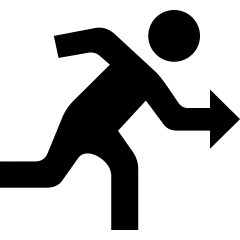Outliers are ‘unusual’ observations: observation quite different (larger or smaller) than the bulk of the data. Deciding whether or not an observation is ‘unusual’ is arbitrary, so ‘rules’ for identifying outliers are somewhat arbitrary too.

Definition 13.10 (Outliers) An outlier is an observation that is ‘unusual’ compared to the bulk of the data (either larger or smaller). Rules for identifying outliers are arbitrary.

Two rules for identifying outliers are:

Understanding the first rule requires studying bell-shaped distributions first. Knowing which rule to use is important.

### 13.5.1 Bell-shaped (normal) distributions and the 68–95–99.7 rule

To begin, identifying outliers will be studied for data approximately symmetrically distributed. More specifically, symmetric distributions with a bell shape will be studied. For example, the heights of husbands in the UK have an approximate bell shape (Fig. 13.8, left panel). Most men are between 160 and 185cm; a few are shorter than 160cm and a few taller than 185cm. More formally, bell-shaped distributions are called normal distributions.

These data are from a sample. Of course, every sample is likely to contain different men, and every sample of men will produce a slightly different histogram.

For convenience then, histograms may be smoothed, so that the smoothing produces a shape that represents an ‘average’ of all these possible sample histograms (in other words, an estimate of how the heights may be distributed in the population). For example, see the animation below. The solid line represents the average of many sample histograms.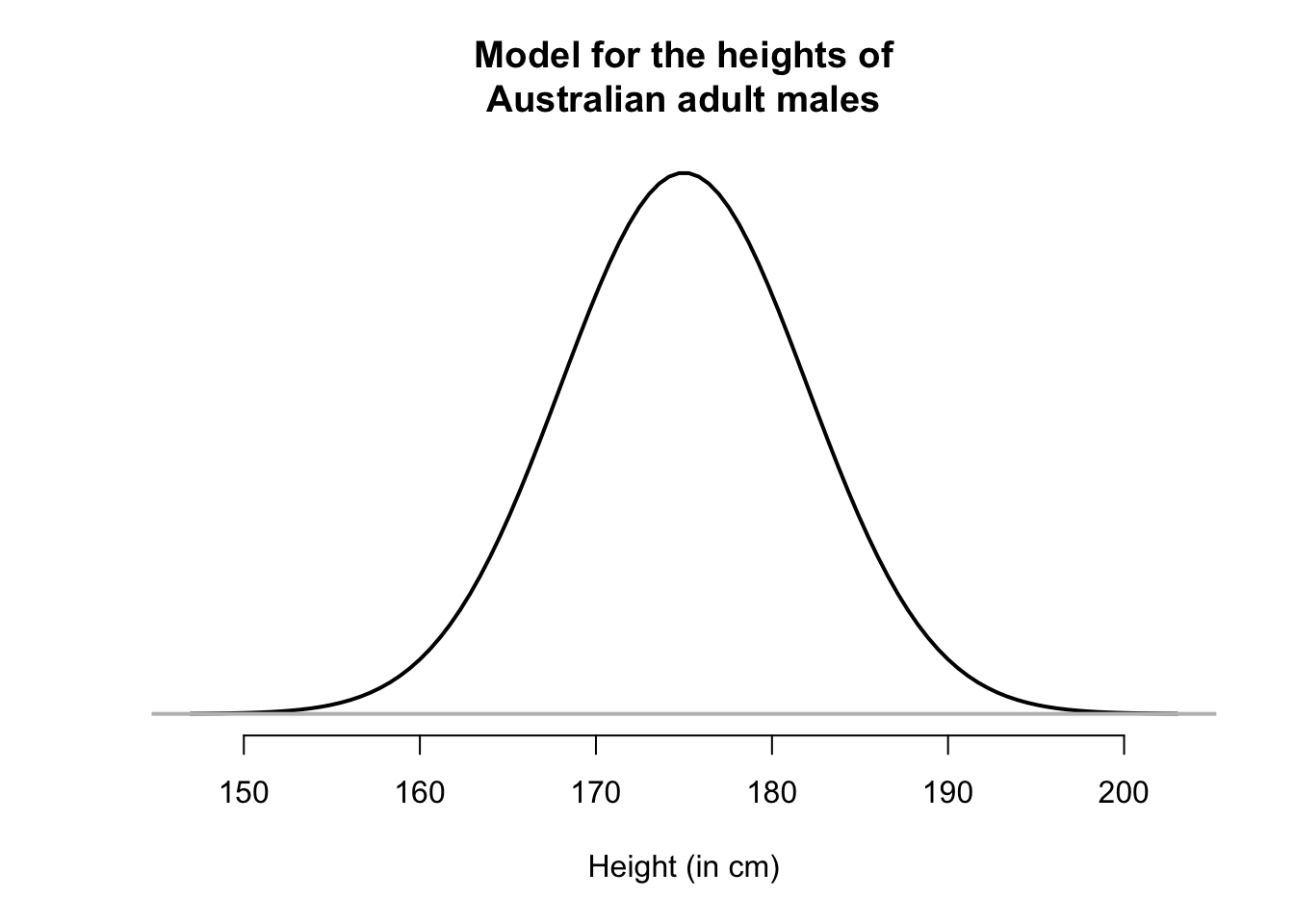The smoothed histogram can be drawn can be considered as representing 100% of the observations; after all, every husband in the sample has a height, so is represented somewhere in the histogram. When we do this, the areas under the normal curve are theoretical percentages of the total number.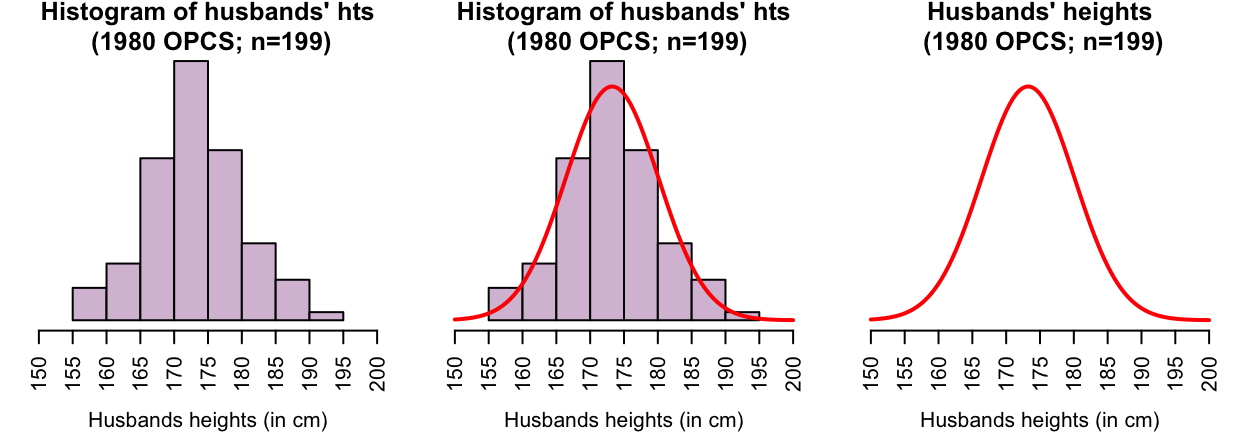FIGURE 13.8: The heights of husbands have an approximate normal distribution

The smoothed histogram represents all of the husbands’ heights (that is, 100%). Using this idea, areas of the histogram can be shaded (Fig. 13.9) to represent various percentages of the husbands’ heights. For example:

• The middle 50% of husbands (Fig. 13.9, centre panel) are between about 168 and 178cm tall.
• The tallest 20% of husbands (Fig. 13.9, right panel) are taller than about 179cm.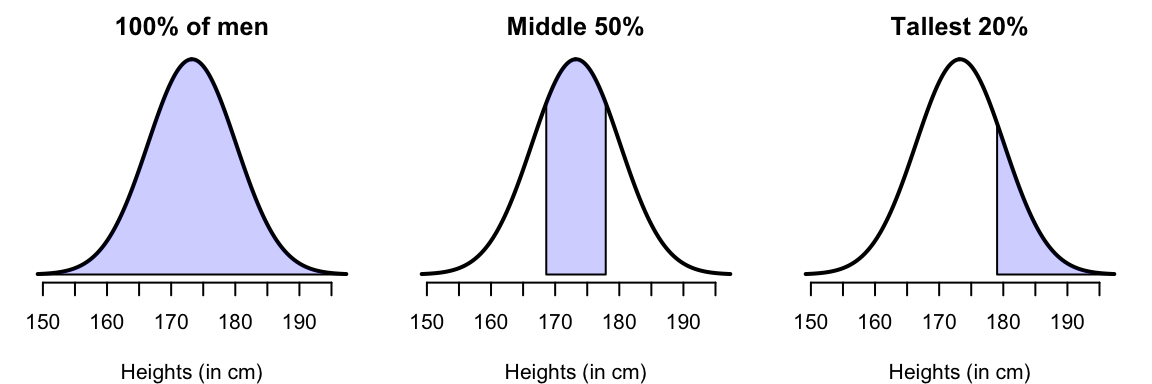FIGURE 13.9: The heights of husbands, with certain percentages shaded

Importantly, for any normal distribution, whatever the mean or standard deviation, the areas under the smoothed curve approximately follow this important rule: The 68–95–99.7 rule.

Definition 13.11 (The 68–95–99.7 Rule (or the Empirical Rule)) For any bell-shaped distribution, approximately:

• 68% of observations lie within one standard deviation of the mean;
• 95% of observations lie within two standard deviations of the mean;
• 99.7% of observations lie within three standard deviations of the mean.
The 68–95–99.7 rule, or the empirical rule, is one of the most important rules we will see.

The animation below shows how the 68–95–99.7 works.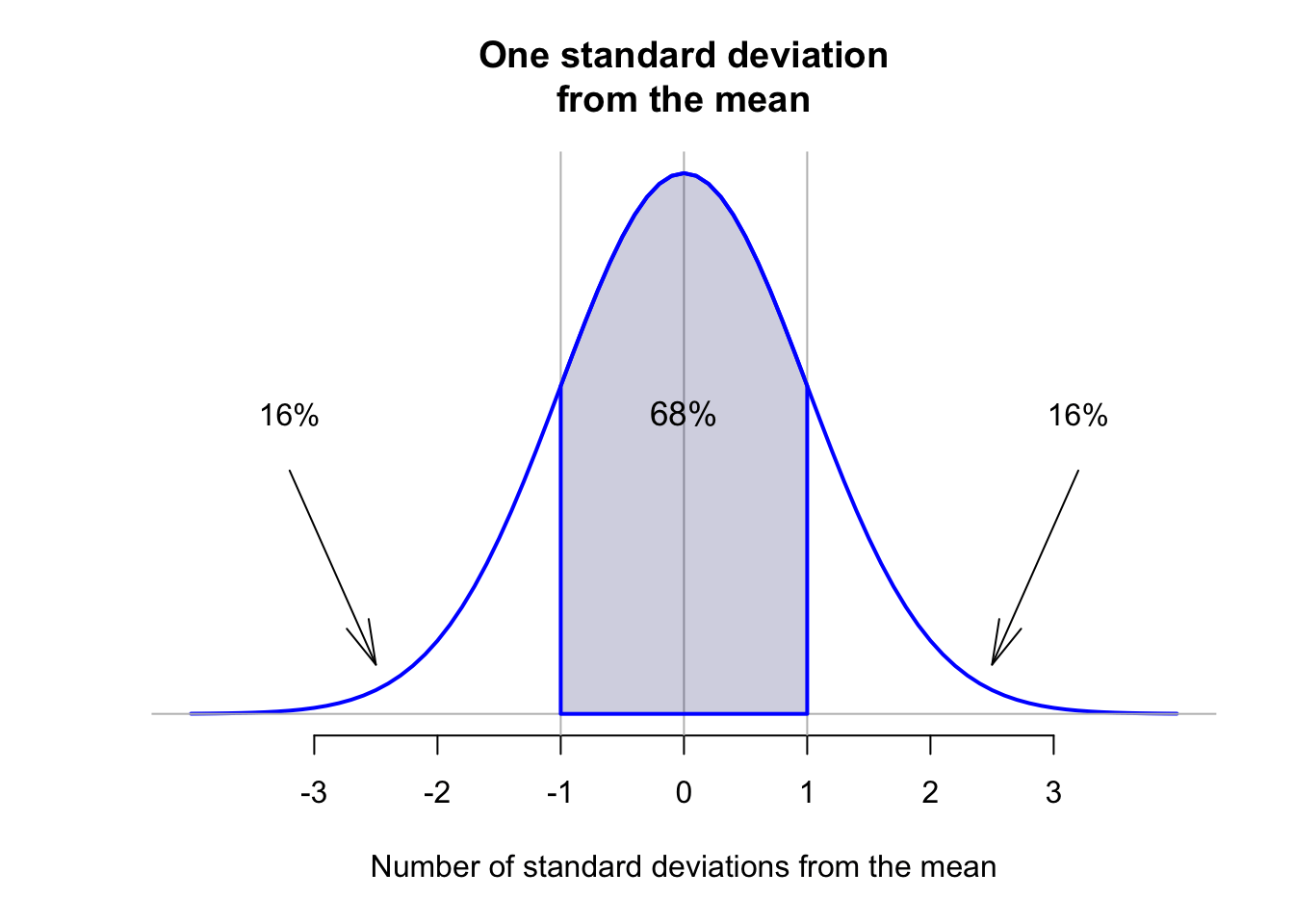The percentages given in the 68–95–99.7 rule are approximate; the exact percentages are 68.27%, 95.45% and 99.73% respectively.

The 68–95–99.7 rule can be used to understand variables that have an approximate normal distribution. For example, consider the heights of husbands again (Fig. 13.10); the sample mean height is $$\bar{x} = 173.2$$cm; the sample standard deviation is $$s = 6.88$$cm. Using the 68–95–99.7 rule, approximately 68% of the husbands would have heights between

• $$173.2 - 6.88 = 166.3$$cm and
• $$173.2 + 6.88 = 180.1$$cm.

(In fact, 71% of husbands in the sample are between 166.3cm and 180.1cm tall, close to the expected 68%.) Similarly, approximately 95% of the husbands would have heights between

• $$173.2 - (2\times 6.88) = 159.4$$cm and
• $$173.2 + (2\times 6.88) = 187.0$$cm.
Think 13.9 (69–95–99.7 rule) For the husbands’ heights, the sample mean height is $$173.2$$cm; the sample standard deviation is $$6.88$$cm. Using the 68–95–99.7 rule, about 99.7% of the husbands are between what heights?
Three standard deviations is $$3\times6.88=20.64$$; about 99.7% of husbands are between $$(173.2 - 20.64) = 152.6$$cm and $$(173.2 + 20.64) = 193.8$$cm.

The empirical rule indicates that 99.7% of observations are within 3 standard deviations of the mean. That is, almost all observations are within three standard deviations of the mean.

This suggests a rule for identifying outliers in approximately bell-shaped distributions: any observation more than 3 standard deviations away from the mean is unusual, so may be considered an outlier. More generally, this rule is often applied to approximately symmetric distributions.

Bell-shaped (normal) distributions are studied further later (for example, Chap. 17).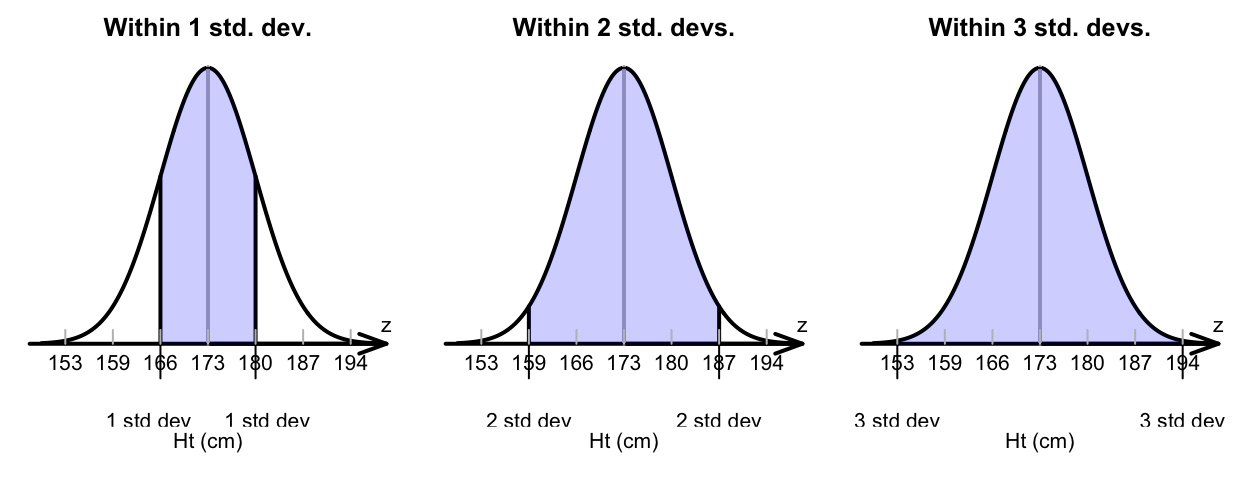FIGURE 13.10: The heights of husbands, showing the 68–95–99.7 rule in use

### 13.5.2 The standard deviation rule for identifying outliers

One rule for identifying outliers is based on the 68–95–99.7 rule.

Definition 13.12 (Standard deviation rule for identifying outliers) For approximately symmetric distributions, an observation more than three standard deviations from the mean may be considered an outlier.

This rule uses the mean and the standard deviation, so this rule is suitable for approximately symmetric distributions (when means and standard deviations are sensible numerical summaries to use). Although this rule is based on normal distributions, it has proved useful for many approximately-symmetric distributions.

All rules for identifying outliers are arbitrary. For example, the standard deviation rule is sometimes given slightly differently; for example, outliers identified as observations more than 2.5 standard deviations away from the mean. Since all rules for identifying outliers are arbitrary, both rules are acceptable.

### 13.5.3 The IQR rule for identifying outliers

Since the standard deviation rule for identifying outliers relies on the mean and standard deviation, it is not appropriate for non-symmetric distributions. Another rule is needed for identifying outliers in these situations: the IQR rule.

Definition 13.13 (IQR rule for identifying outliers) The IQR rule identifies mild and extreme outliers as:

• Extreme outliers: observations $$3\times \text{IQR}$$ more unusual than $$Q_1$$ or $$Q_3$$.

• Mild outliers: observations $$1.5\times \text{IQR}$$ more unusual than $$Q_1$$ or $$Q_3$$ (that are not also extreme outliers).

This definition is much easier to understand using an example.

Example 13.10 (IQR rule for identifying outliers) An engineering project studied a new building material, to estimate the average permeability.

Measurements of permeability time (the time for water to permeate the sheets) were taken from 81 pieces of material (in seconds). For these data $$Q_1 = 24.7$$ and $$Q_3 = 50.6$$, so we find that $$\text{IQR} = {50.6 - 24.7 = 25.9}$$. Then, extreme outliers observations are $$3\times 25.9 = 77.7$$ more unusual than $$Q_1$$ or $$Q_3$$. That is, extreme outliers are observations:

• more unusual than $$24.7 - 77.7 = -53.0$$ (that is, less than $$-53$$); or
• more unusual than $$50.6 + 77.7 = 128.3$$ (that is, greater than $$128.3$$).

Mild outliers observations are $$1.5\times 25.9 = 38.9$$ more unusual than $$Q_1$$ or $$Q_3$$ (that are not also extreme outliers). That is, mild outliers are

• more unusual than $$24.7 - 38.9 = -14.2$$ (that is, less than $$-14.2$$); or
• more unusual than $$50.6 + 38.9 = 89.5$$ (that is, greater than $$89.5$$).
The outliers are identified when constructing a boxplot: the ‘whiskers’ extended to the most extreme observation remaining after excluding mild and extreme observations; then, mild outliers are shown using a $$\circ$$, and extreme outliers are shown using a $$\star$$.

You don’t need to do this (that’s what software is for), but you do need to understand what the software is doing. Construction of the boxplot is shown in the animation below.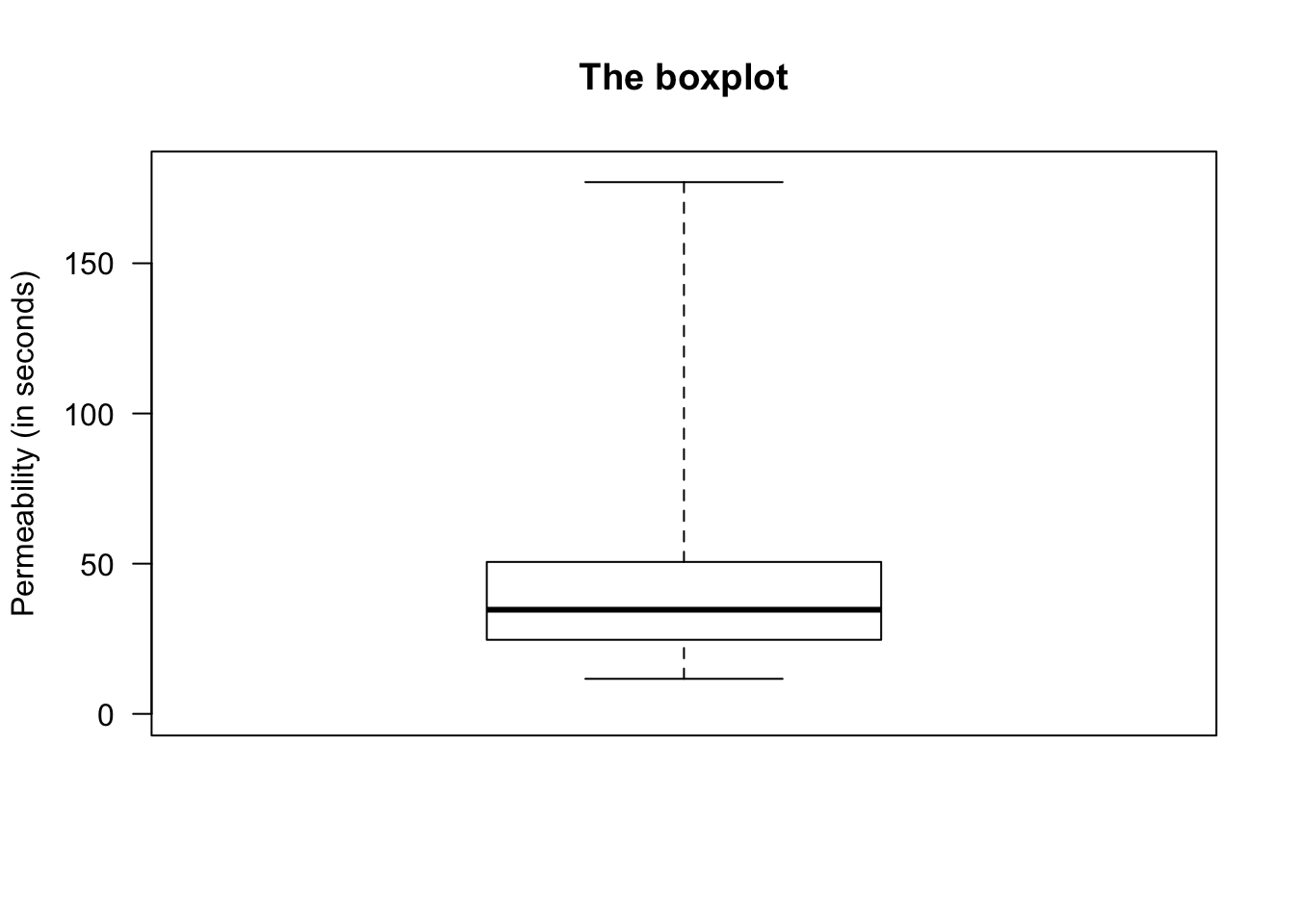### 13.5.4 When to use which rule?

In summary, two common ways to identify outliers are:

• For approximately symmetric distributions: use the standard deviation rule.
• For any distribution, but primarily for those skewed or with outliers: use the IQR rule.

But remember: All rules for identifying outliers are arbitrary!

Example 13.11 (Boxplots and histograms) For the permeability data , compare the boxplot and histogram (Fig. 13.11). Can you see how the boxplot identifies the observations in the histogram that seem to be outliers?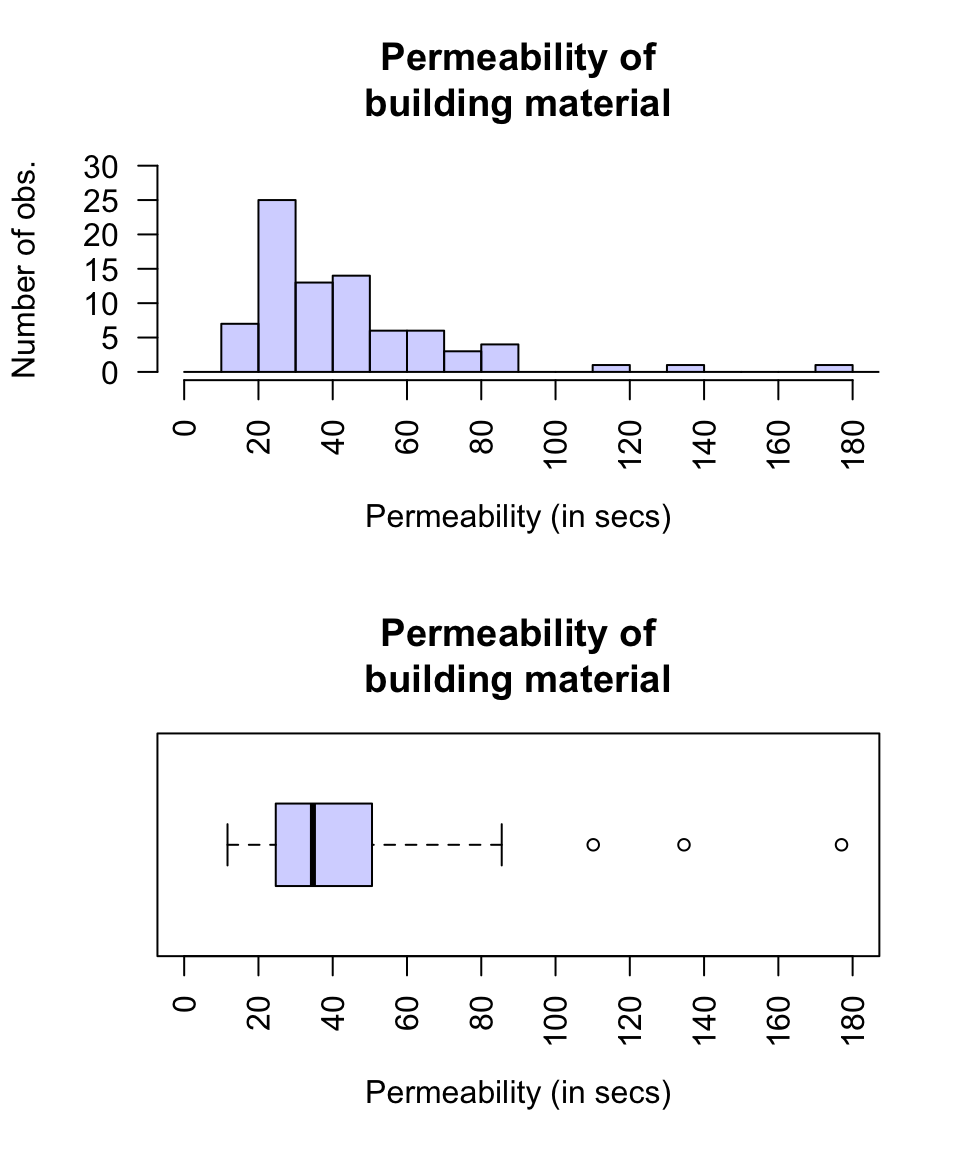FIGURE 13.11: A boxplot and histogram for the permeability data

Think 13.10 (Understanding data summaries) In an American study , the lung capacity (FEV) of youth aged 3 to 19 was measured. The data are slightly skewed right, and the average FEV is about 2.6 litres, The FEV varies from about 0.8 to 5.8 litres, with no outliers.

Using this information, sketch the boxplot and the histogram for the data.

### References

Badiou A, Marsh C, Gauchet M. Exploring data: An introduction to data analysis for social scientists. Cambridge, UK: Polity Press; 1988.
Hald A. Statistical theory with engineering applications. New York: John Wiley; Sons; 1952.
Hand DJ, Daly F, Lunn AD, McConway KY, Ostrowski E. A handbook of small data sets. London: Chapman; Hall; 1996.
Tager IB, Weiss ST, Rosner B, Speizer FE. Effect of parental cigarette smoking on the pulmonary function of children. American Journal of Epidemiology. 1979;110(1):15–26.